# YOLOv7来临：图解网络结构模块

【前言】此篇续上篇论文解读《YOLOv7来临：论文解读附代码解析》，对YOLOv7网络结构中的一些重要模块进行讲解。

### 一、ReOrg 操作¶

class ReOrg(nn.Module):
def __init__(self):
super(ReOrg, self).__init__()

def forward(self, x):  # x(b,c,w,h) -> y(b,4c,w/2,h/2)
return torch.cat([x[..., ::2, ::2], x[..., 1::2, ::2], x[..., ::2, 1::2], x[..., 1::2, 1::2]], 1)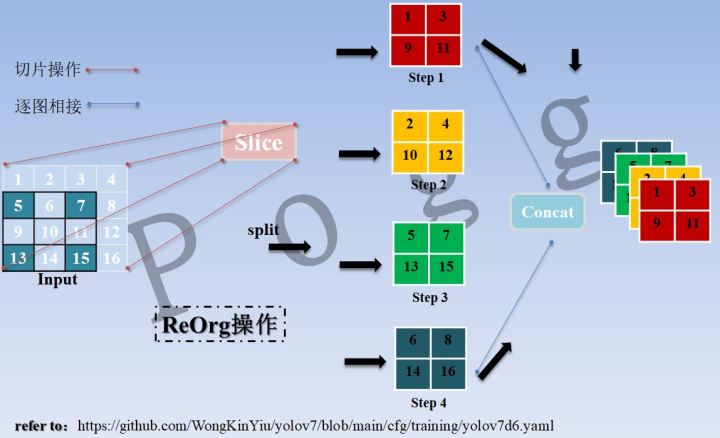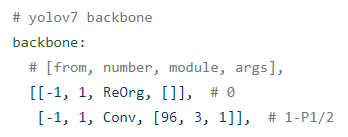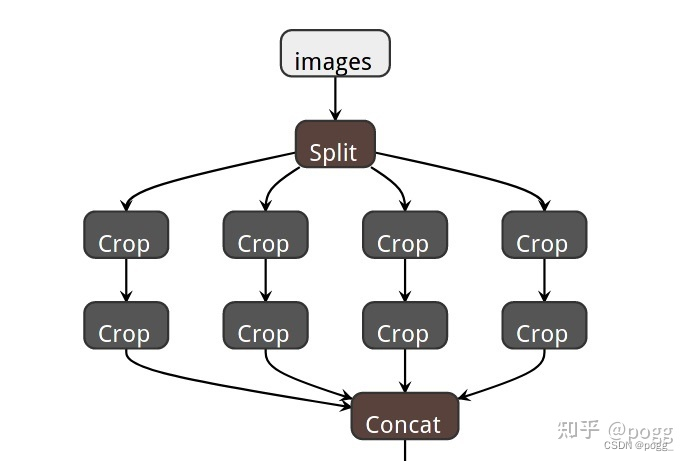### 二、多路卷积模块¶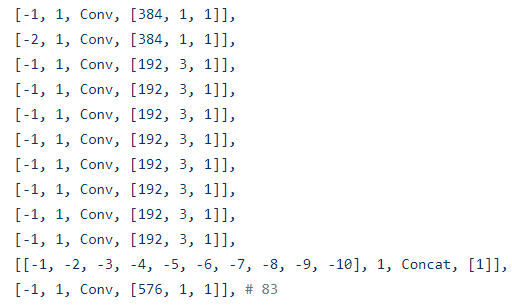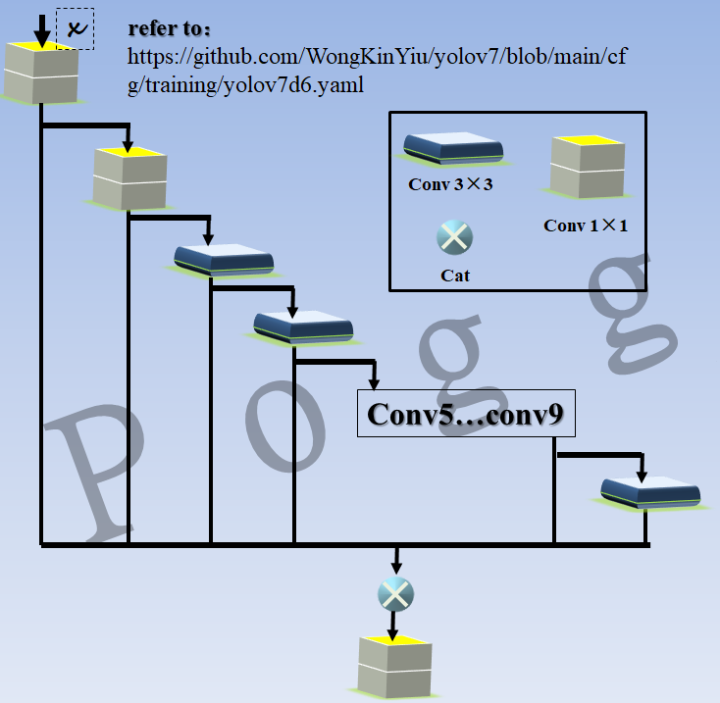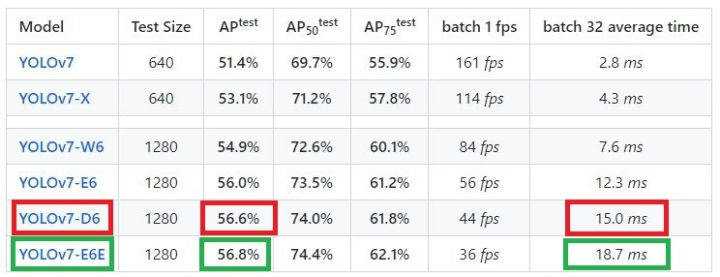### 三、DownC模块¶

• 对于定义一，将kernel_size和stride设为2的MaxPool2d称为MP；

class MP(nn.Module):
def __init__(self, k=2):
super(MP, self).__init__()
self.m = nn.MaxPool2d(kernel_size=k, stride=k)

def forward(self, x):
return self.m(x)

class SP(nn.Module):
def __init__(self, k=3, s=1):
super(SP, self).__init__()
self.m = nn.MaxPool2d(kernel_size=k, stride=s, padding=k // 2)

def forward(self, x):
return self.m(x)


class DownC(nn.Module):
# Spatial pyramid pooling layer used in YOLOv3-SPP
def __init__(self, c1, c2, n=1, k=2):
super(DownC, self).__init__()
c_ = int(c1)  # hidden channels
self.cv1 = Conv(c1, c_, 1, 1)
self.cv2 = Conv(c_, c2//2, 3, k)
self.cv3 = Conv(c1, c2//2, 1, 1)
self.mp = nn.MaxPool2d(kernel_size=k, stride=k)

def forward(self, x):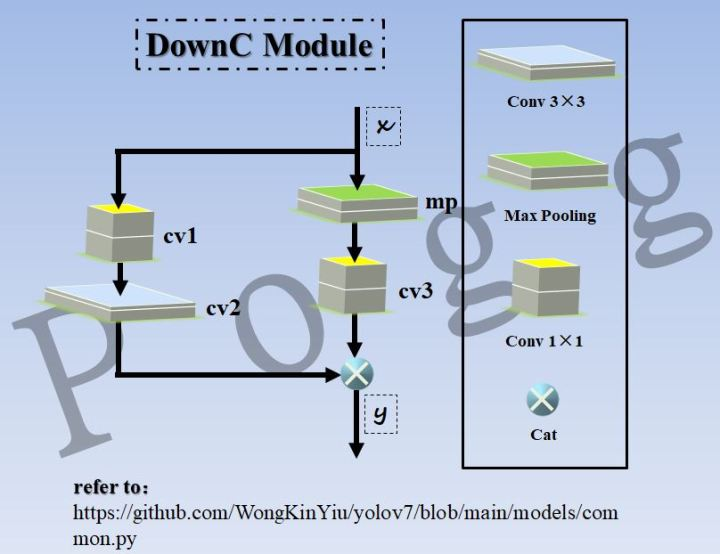### 四、SPPCSPC模块¶

class SPPCSPC(nn.Module):
# CSP https://github.com/WongKinYiu/CrossStagePartialNetworks
def __init__(self, c1, c2, n=1, shortcut=False, g=1, e=0.5, k=(5, 9, 13)):
super(SPPCSPC, self).__init__()
c_ = int(2 * c2 * e)  # hidden channels
self.cv1 = Conv(c1, c_, 1, 1)
self.cv2 = Conv(c1, c_, 1, 1)
self.cv3 = Conv(c_, c_, 3, 1)
self.cv4 = Conv(c_, c_, 1, 1)
self.m = nn.ModuleList([nn.MaxPool2d(kernel_size=x, stride=1, padding=x // 2) for x in k])
self.cv5 = Conv(4 * c_, c_, 1, 1)
self.cv6 = Conv(c_, c_, 3, 1)
self.cv7 = Conv(2 * c_, c2, 1, 1)

def forward(self, x):
x1 = self.cv4(self.cv3(self.cv1(x)))
y1 = self.cv6(self.cv5(torch.cat([x1] + [m(x1) for m in self.m], 1)))
y2 = self.cv2(x)
return self.cv7(torch.cat((y1, y2), dim=1))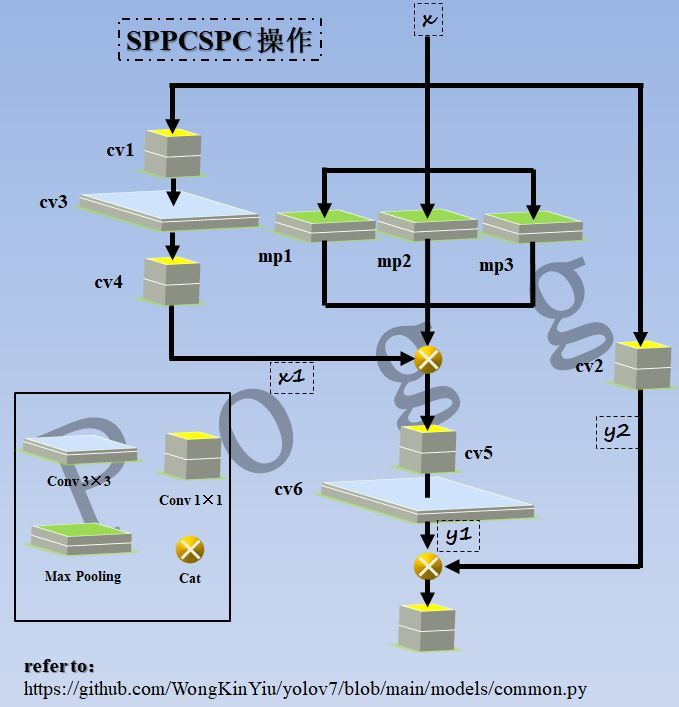### 五、RepConv模块¶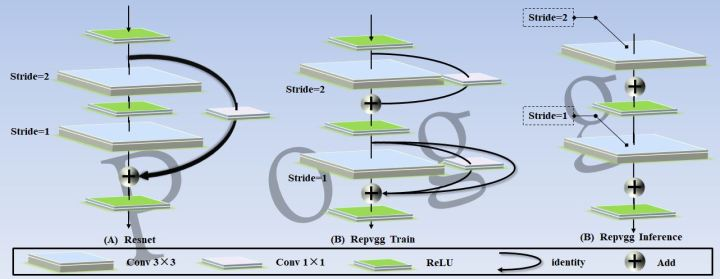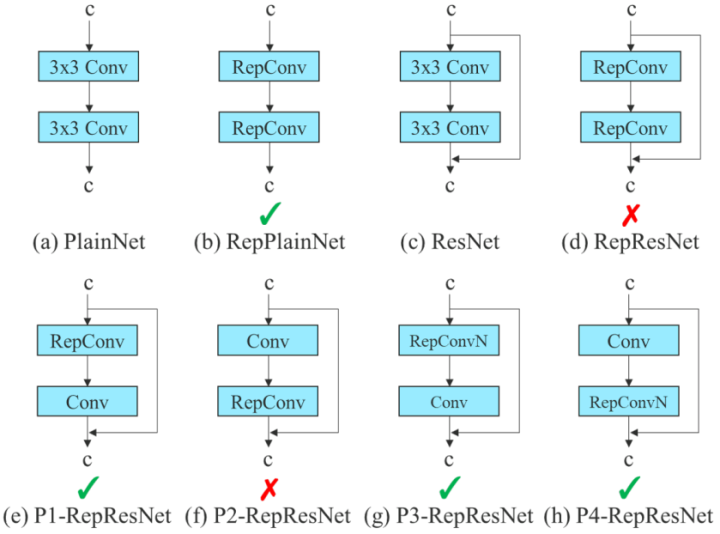### E-ELAN模块¶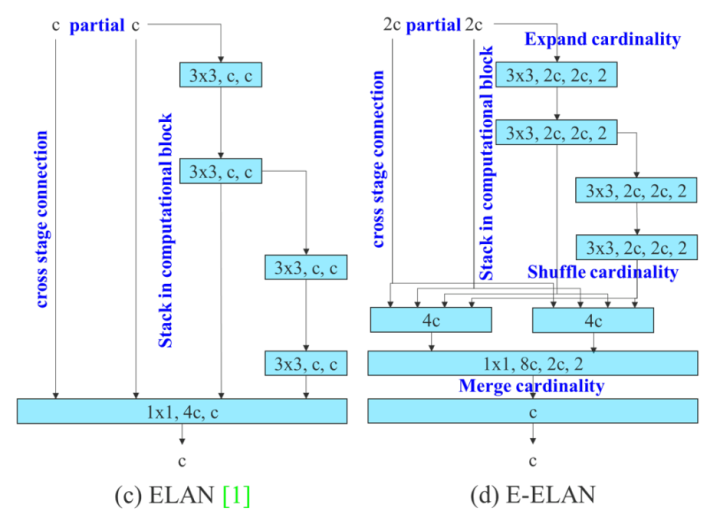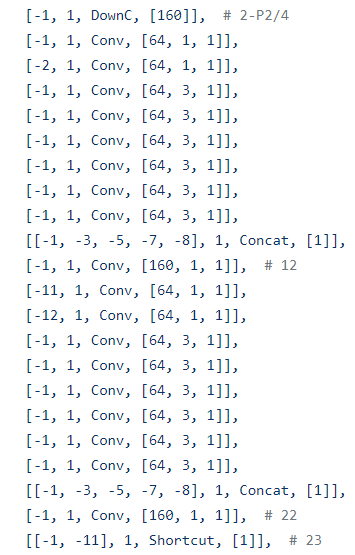### 总结¶

• 论文中提到的SiLU函数在官方仓库中并未见到
• YOLOv7-Tiny目前仅提供了SiLU版本，未提供论文中的ReLU版本
• 官方仓库所使用的激活函数统一为LeakyReLU
• 目前暂未在仓库中找到论文中提到的merge cardinality和shuffle cardinality等操作
• 作者在论文提及多分支结构的弊端，但在模型中依旧使用类似densenet多分支，应该是实验出该模型在gpu架构上高效，但训练以及在低算力平台（此处指量化4bit后的版本)的速度问题有待商榷。